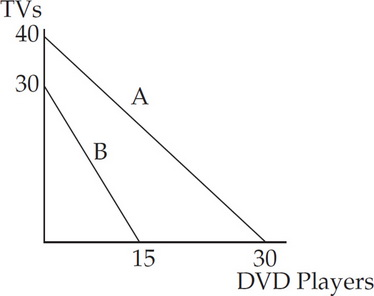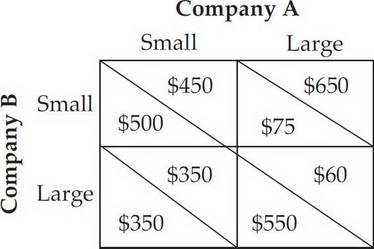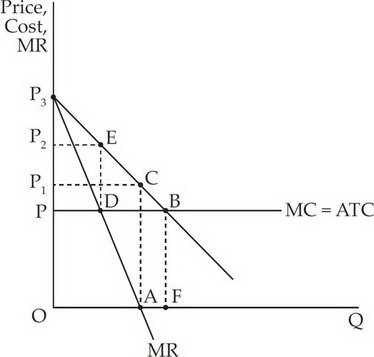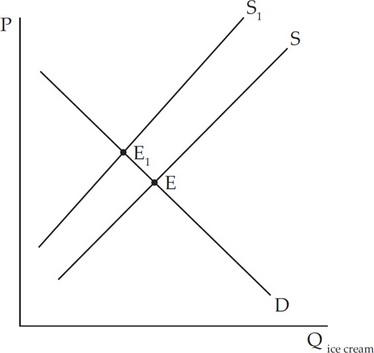# AP Microeconomics Practice Test: Factor Markets

### Test Information19 questions23 minutes

1. A competitive firm's demand for labor is determined directly by

2. Relative to a competitive input market, a monopsony

3. Which of the following is not among the methods unions use to increase wages?

4. A bilateral monopoly exists when

5. Because people with relatively low incomes spend a larger percentage of their income on food than people with relatively high incomes, a sales tax on food would fall into which category of taxes?

6. Assume a firm hires labor for \$15 each and sells its products for \$3 each. If the MP of the 3rd worker is 10, which of the following statements would be the most true?

7. If the government regulates a monopoly to produce at the allocative efficient quantity, which of the following would be true?

8. Marginal revenue equals marginal cost at the point where

9. If the government subsidizes producers in a perfectly competitive market, then

10. A price discriminating monopoly differs from a non-discriminating monopoly because a discriminating monopoly

11. Which of the following could have caused an increase in the demand for ice cream cones?

12.The above diagram represents Country A and B's production possibilities with the use of the same resources. Which of the following would be true for Country A and B?

13. A student eats 3 slices of pizza while studying for his Economics exam. The marginal utility of the first slice of pizza is 10 utils, the second slice is 7 utils, and the third slice is 3 utils. Which of the statements below holds true with the above data?

14. If corn is produced in a perfectly competitive market and the government placed a price ceiling above equilibrium, which of the following would be true?

15. Which of the following examples would result in consumers paying for the largest burden of an excise tax placed on a producer?

16.Company A and B above operate in a non-collusive oligopolistic market. Each need to decide whether to have a large or small advertising strategy. According to the data, the dominant strategy for Company A and B would be

17. A monopoly is less efficient than a perfect competitor because

18.For this monopolist profit is the area represented by

19.In the graph above, the shift in the supply curve from S to S1 for ice cream could occur because